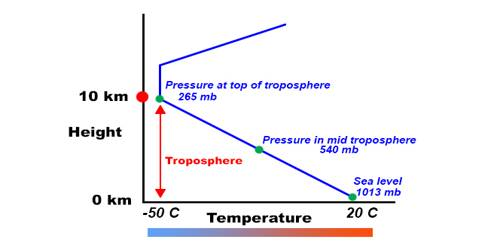Geology

# Vertical Variation of Pressure in Earth SurfaceVertical Variation of Pressure in Earth Surface

In the lower atmosphere, the pressure decreases rapidly with height. The decrease amounts to about 1 m for each 10 m increase in elevation. It does not always decrease at the same rate. The table gives the average pressure and temperature at selected levels of elevation for a standard atmosphere.

Table: Standard Pressure and Temperature at Selected LevelsA relatively simple version of the vertical fluid pressure variation is simply that the pressure difference between two elevations is the product of elevation change, gravity, and density. The vertical variation is particularly important, as it results from the pull of gravity on the fluid. For the same given fluid, a decrease in elevation within it corresponds to a taller column of fluid weighing down on that point. Vertical pressure variation of the Atmosphere of Earth depends on air density.

The vertical pressure gradient force is much larger than that of the horizontal pressure gradient. But, it is generally balanced by a nearly equal but opposite gravitational force. Hence, we do not experience strong upward winds.